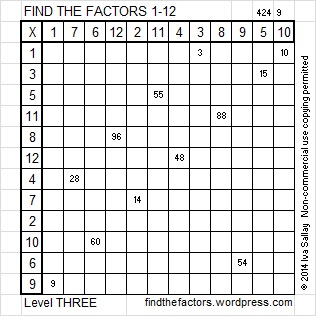# Two Factor Pairs of 1716 Make Sum-Difference!

### Today’s Puzzle:

How many factor pairs does 1716 have? Twelve. The factors in one of those factor pairs add up to 145 and the factors in a different one subtract to 145. If you can find those two factor pairs, then you can solve this puzzle!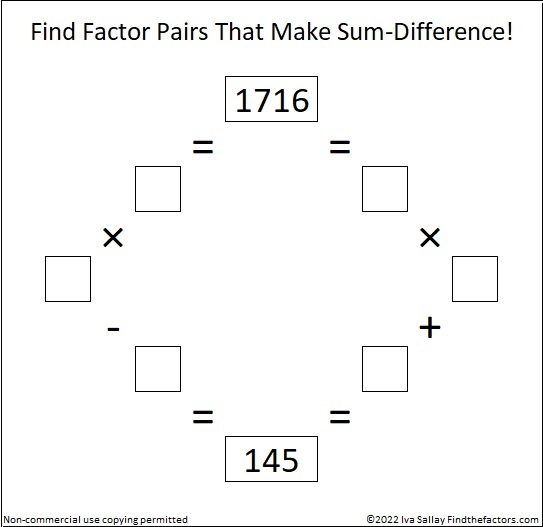Try to solve it all by yourself, but if you need some help, you can scroll down to learn more about the factors of 1716.

### A Factor Tree for 1716:

3 is a factor of 1716 because 1 + 7 + 1 + 6 = 15, a multiple of 3.
11 is a factor of 1716 because 1 – 7 + 1 – 6 = -11.

3 ·11 is 33. Here is one of MANY possible factor trees for 1716. This one uses the fact that 33 · 52 = 1716: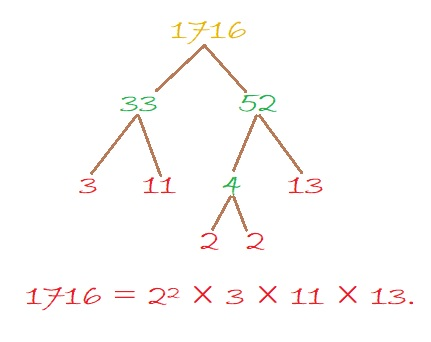### Factors of 1716:

• 1716 is a composite number.
• Prime factorization: 1716 = 2 × 2 × 3 × 11 × 13, which can be written 1716 = 2² × 3 × 11 × 13.
• 1716 has at least one exponent greater than 1 in its prime factorization so √1716 can be simplified. Taking the factor pair from the factor pair table below with the largest square number factor, we get √1716 = (√4)(√429) = 2√429.
• The exponents in the prime factorization are 2, 1, 1, and 1. Adding one to each exponent and multiplying we get (2 + 1)(1 + 1)(1 + 1)(1 + 1) = 3 × 2 × 2 × 2 = 24. Therefore 1716 has exactly 24 factors.
• The factors of 1716 are outlined with their factor pair partners in the graphic below.Here’s a chart of those same factor pairs but in reverse order with their sums and differences included.### More About the Number 1716:

1716 is the hypotenuse of a Pythagorean triple:
660-1584-1716, which is (5-12-13) times 132.

OEIS.org reminds us that 1716 is in the 6th and 7th columns of the 13th row of Pascal’s Triangle: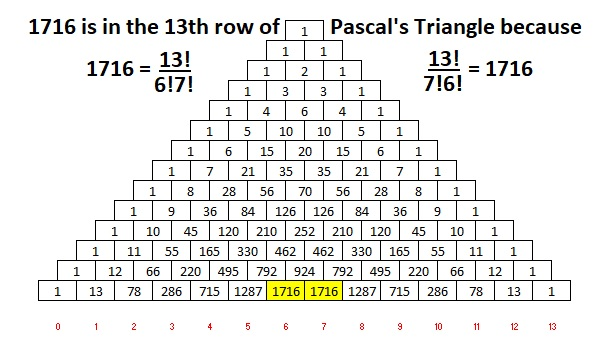# Find the Factor Pairs of 1710. Which One Sums to 181? Which One Subtracts to 181?

### Today’s Puzzle:

1710 has TWELVE factor pairs. Two of those factor pairs are special. If you add up the factors in one of them, you will get 181, but get this, if you subtract the factors in the other one, you will also get 181. Find the two factor pairs that do that, and you will have essentially solved this puzzle:This Sum-Difference puzzle would be helpful if you had to factor any of these trinomials:
45x² – 181x + 38,
95x² – 181x – 18,
90x² + 181x + 19,
38x² + 181x – 45,

The trinomials are in the form ax² + bx + c, where b = 181, and a·c = ±1710. Whether the 1710 is positive or negative makes all the difference in the world when factoring them!

### Factors of 1710:

• 1710 is a composite number.
• Prime factorization: 1710 = 2 × 3 × 3 × 5 × 19, which can be written 1710 = 2 × 3² × 5 × 19.
• 1710 has at least one exponent greater than 1 in its prime factorization so √1710 can be simplified. Taking the factor pair from the factor pair table below with the largest square number factor, we get √1710 = (√9)(√190) = 3√190.
• The exponents in the prime factorization are 1, 2, 1, and 1. Adding one to each exponent and multiplying we get (1 + 1)(2 + 1)(1 + 1)(1 + 1) = 2 × 3 × 2 × 2 = 24. Therefore 1710 has exactly 24 factors.
• The factors of 1710 are outlined with their factor pair partners in the graphic below.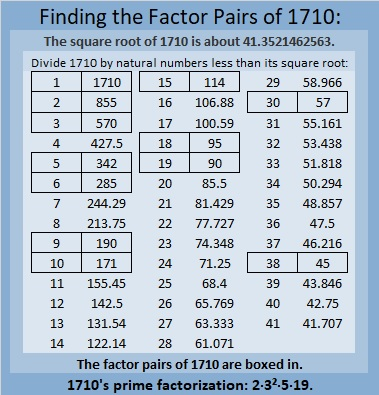The following chart lists 1710’s factor pairs with their sums and differences: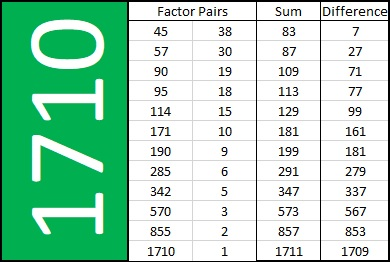### More About the Number 1710:

1710 is the hypotenuse of a Pythagorean triple:
1026-1368-1710, which is (3-4-5) times 342.

# Math Happens When Factors of 1620 Make Sum-Difference

### Math Happens Puzzle:

Math Happens put another one of my puzzles in the Austin Chronicle and the Orange Leader!

You can see the puzzle on page 23 of this e-edition or this pdf of the newspaper. You can also find links to all of my Sum-Difference puzzles here.

Here is another one of Math Happen’s amazing puzzles. The tabletops shown are exactly the same. Click on it to see proof!

Math Happens in many different ways as you can see in their blog post from February 5. You can also look for Math Happens on a page in the middle of each of these  2020 issues or 2021 issues of the Austin Chronicle newspaper online.

Would you like puzzles like these in your community newspaper? Have your paper contact Math Happens on Twitter and make it happen!

### Today’s Sum-Difference Puzzles:

Just like the number 6 in the newspaper puzzle above, 180 and 1620 both have factor pairs that make sum-difference. To help you solve these puzzles, I’ve listed all of their factor pairs in the graphics below the puzzle.As shown below, 180 has nine factor pairs. One of those pairs adds up to 41, and another one subtracts to 41. Put the factors in the appropriate boxes in the first puzzle.The needed factors for the second puzzle are multiples of the numbers in the first puzzle. 1620 has fifteen factor pairs. One of the factor pairs adds up to ­123, and a different one subtracts to 123. If you can identify those factor pairs, then you can solve the second puzzle!

### What Are the Factors of 1620?• 1620 is a composite number.
• Prime factorization: 1620 = 2 × 2 × 3 × 3 × 3 × 3 × 5, which can be written 1620 = 2² × 3⁴ × 5.
• 1620 has at least one exponent greater than 1 in its prime factorization so √1620 can be simplified. Taking the factor pair from the factor pair table below with the largest square number factor, we get √1620 = (√324)(√5) = 18√5.
• The exponents in the prime factorization are 4, 2 and 1. Adding one to each exponent and multiplying we get (2 + 1)(4 + 1)(1 + 1) = 3 × 5 × 2 = 30. Therefore 1620 has exactly 30 factors.
• The factors of 1620 are outlined with their factor pair partners in the graphic above.

### Factor Trees for 1620:

Here are two of the MANY possible factor trees for 1620: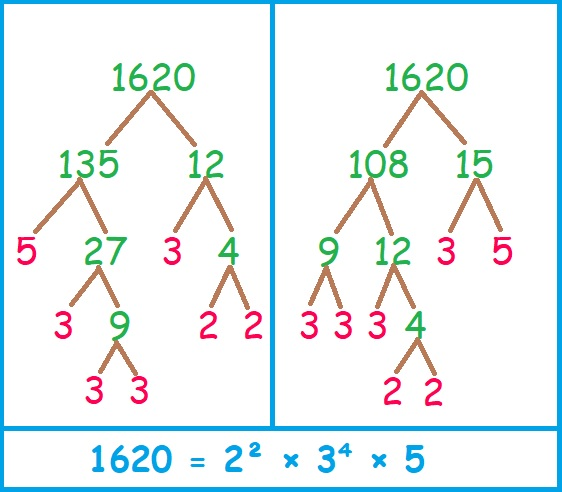Hint: I chose to build these factor trees with those two sets of factor pairs for a reason.

### More about the Number 1620:

1620 is the sum of the interior angles of a hendecagon (11-sided polygon) because
(11 – 2)180 = 1620.1620 is the sum of two squares:
36² + 18² = 1620.

1620 is the hypotenuse of a Pythagorean triple:
972-1296-1620, calculated from 36² – 18², 2(36)(18), 36² + 18².
It is also (3-4-5) times 324.

This is only some of the math that happens with the number 1620.

# Why Do Factor Pairs of 1560 Make Sum-Difference?

### Today’s Puzzle:

1560 has 16 different factor pairs. One of those pairs sum up to 89, and another pair subtracts to 89. It is only the 50th time that the sum of a factor pair of a number equals the difference of one of its other factor pairs.

You may have seen other Sum-Difference Puzzles where I’ve paired one puzzle with another puzzle and mentioned that the second puzzle was really the first puzzle in disguise. That is not the case for this puzzle because 89 is a prime number. This puzzle is a primitive; there is not a simpler puzzle that is its equivalent. Don’t let that worry you, however, everything you need to solve this puzzle can be found in this post.Although I am making a big deal about our number 1560, it is the 89 which allows this puzzle to exist in the first place. You see, 89 is the hypotenuse of a Pythagorean triple, (39-80-89), and thus, 39² + 80² = 89². Since that triple is a primitive, the sum-difference is a primitive as well.

Note that (40)(39) = 1560.
We want to use the quadratic formula to solve 40x² + 89x + 39 = 0 and 40x² + 89x – 39 = 0. Let’s combine the left sides of those two equations into one expression:
40x² + 89x ± 39.
The discriminant would be 89² – 4(40)(±39)
= 89² ± 4(40)(39)
= 89² ± 2(80)(39)
= 39² + 80² ± 2(80)(39), ( That’s because 39² + 80² = 89².)
= 80² ± 2(80)(39) + 39²
= (80 ± 39)², a perfect square!

That perfect square makes 1560 one of those relatively rare numbers with factor pairs that make sum-difference.

### Factors of 1560:

• 1560 is a composite number.
• Prime factorization: 1560 = 2 × 2 × 2 × 3 × 5 × 13 , which can be written 1560 = 2³ × 3 × 5 × 13.
• 1560 has at least one exponent greater than 1 in its prime factorization so √1560 can be simplified. Taking the factor pair from the factor pair table below with the largest square number factor, we get √1560 = (√4)(√390) = 2√390.
• The exponents in the prime factorization are 2, 1, and 2. Adding one to each exponent and multiplying we get (3 + 1)(1 + 1)(1 + 1) (1 + 1) = 4 × 2 × 2 × 2 = 32. Therefore 1560 has exactly 32 factors.
• The factors of 1560 are outlined with their factor pair partners in the graphic below.That’s a lot of factor pairs for one number! Here’s a graphic showing those same factor pairs but with their sums and differences included: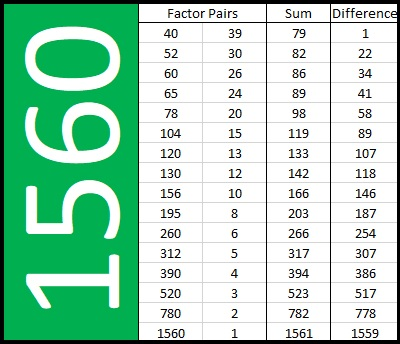Find the 89 in the Sum column and the 89 in the Difference column, and you will see the factor pairs by those 89’s that make the sum and difference needed to solve the puzzle.

### More about the Number 1560:

1560 = 39 × 40, so 1560 is the sum of the first 39 even numbers:
2 + 4 + 6 + 8 + . . . + 74 + 76 + 78 = 1560.

1560 is the hypotenuse of FOUR Pythagorean triples:
384-1512-1560, which is 24 times (16-63-65),
600-1440-1560, which is (5-12-13) times 120,
792-1344-1560, which is 24 times (33-56-65), and
936-1248-1560, which is (3-4-5) times 312.

Since 1560 is a hypotenuse four different ways, could it be the bottom part of four different Sum-Difference puzzles? Yes!

You can find the top part of the puzzle by finding one half of the product of the first two numbers in each triple:
384 × 1512 ÷ 2 = ____________,
600 × 1440 ÷ 2 = ____________,
792 × 1344 ÷ 2 = ____________,
936 × 1248 ÷ 2 = ____________.

The numbers that go in the blanks are all between 100 thousand and one million, but each one of those numbers will have a factor pair that adds up to 1560 as well as another one that subtracts to 1560. If you find them, go ahead and brag about it! It will be quite an accomplishment!

### A Factor Tree for 1560:

Here is one of MANY possible factor trees for 1560: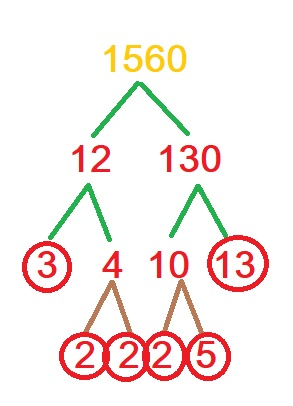# Factors of 1536 Make Sum-Difference!

### Today’s Puzzles:

1536 has 10 different factor pairs. One of those pairs adds up to 80 and a different one subtracts to give 80. Can you find the factor pairs that make sum-difference and write them in the puzzle? You can look at all of the factor pairs of 1536 in the graphic after the puzzle, but the second puzzle is really just the first puzzle in disguise. So try solving that easier puzzle first.That first puzzle is the first possible Sum-Difference puzzle. The second puzzle is only the 49th possible puzzle.

### Factors of 1536:

• 1536 is a composite number.
• Prime factorization: 1536 = 2 × 2 × 2 × 2 × 2 × 2 × 2 × 2 × 2 × 3, which can be written 1536 = 2⁹ × 3.
• 1536 has at least one exponent greater than 1 in its prime factorization so √1536 can be simplified. Taking the factor pair from the factor pair table below with the largest square number factor, we get √1536 = (√256)(√6) = 16√6.
• The exponents in the prime factorization are 9 and 1. Adding one to each exponent and multiplying we get (9 + 1)(1 + 1) = 10 × 2 = 20. Therefore 1536 has exactly 20 factors.
• The factors of 1536 are outlined with their factor pair partners in the graphic below.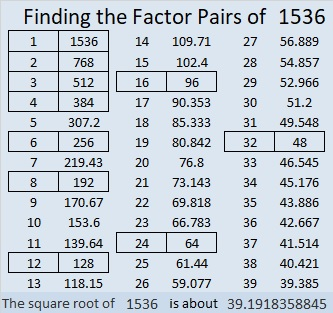### More About the Number 1536:

1536 is the difference of two squares in EIGHT different ways!
385² – 383² = 1536,
194² – 190² = 1536,
131² – 125² = 1536,
100² – 92² = 1536,
70² – 58² = 1536,
56² – 40² = 1536,
44² – 20² = 1536, and
40² – 8² = 1536.

1536 = 6 × 256.
256 is the 100th number whose square root can be simplified.
1536 is the 600th number whose square root can be simplified.

Here are the 501st to the 600th simplifiable square roots. If at least three square roots are consecutive, they are highlighted.# Celebrating 1500 with a Horse Race and Much More!

### Pick Your Pony!

Writing 1500 posts is quite a milestone. I’ll begin the celebration with an exciting horse race! Let me explain:

Each prime number has exactly 2 factors. Every composite number between 1401 and 1500 has somewhere between 4 and 36 factors. Which quantity of factors do you think will appear most often for these numbers? Pick that amount as your pony and see how far it gets in this horse race!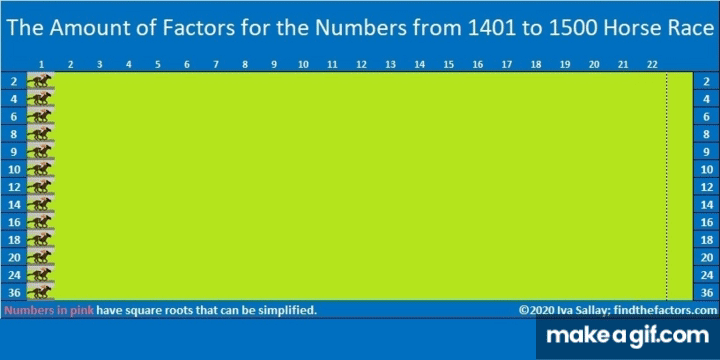make science GIFs like this at MakeaGif

Did the race results surprise you? They surprised me!

### Prime Factorization for numbers from 1401 to 1500:

Here’s a chart showing the prime factorization of all those numbers and the amount of factors each number has. Numbers in pink have exponents in their prime factorization so their square roots can be simplified:### Today’s Puzzles:

Let’s continue the celebration with a puzzle: 1500 has 12 different factor pairs. One of those pairs adds up to 85 and one of them subtracts to give 85. Can you find those factor pairs that make sum-difference and write them in the puzzle? You can look at all of the factor pairs of 1500 in the graphic after the puzzle, but the second puzzle is really just the first puzzle in disguise. So try solving that easier puzzle first.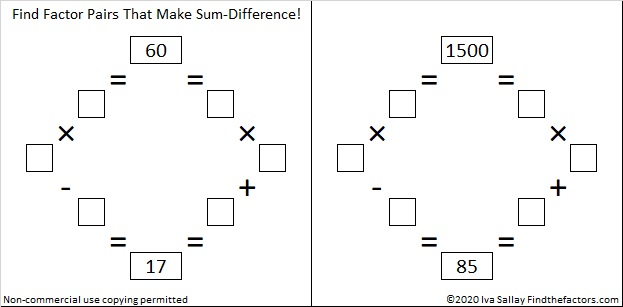### Factors of 1500:

• 1500 is a composite number.
• Prime factorization: 1500 = 2 × 2 × 3 × 5 × 5 × 5, which can be written 1500 = 2² × 3 × 5³
• 1500 has at least one exponent greater than 1 in its prime factorization so √1500 can be simplified. Taking the factor pair from the factor pair table below with the largest square number factor, we get √1500 = (√100)(√15) = 10√15
• The exponents in the prime factorization are 2, 1, and 3. Adding one to each exponent and multiplying we get (2 + 1)(1 + 1)(3 + 1) = 3 × 2 × 4 = 24. Therefore 1500 has exactly 24 factors.
• The factors of 1500 are outlined with their factor pair partners in the graphic below.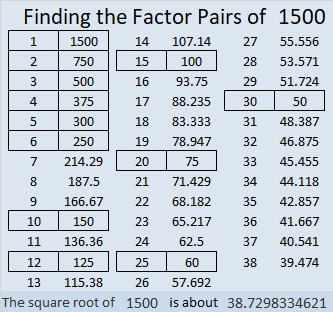### A Forest of Factor Trees:

Take a few minutes to hike in this forest featuring a few of the MANY possible factor trees for 1500. Celebrate each tree’s uniqueness!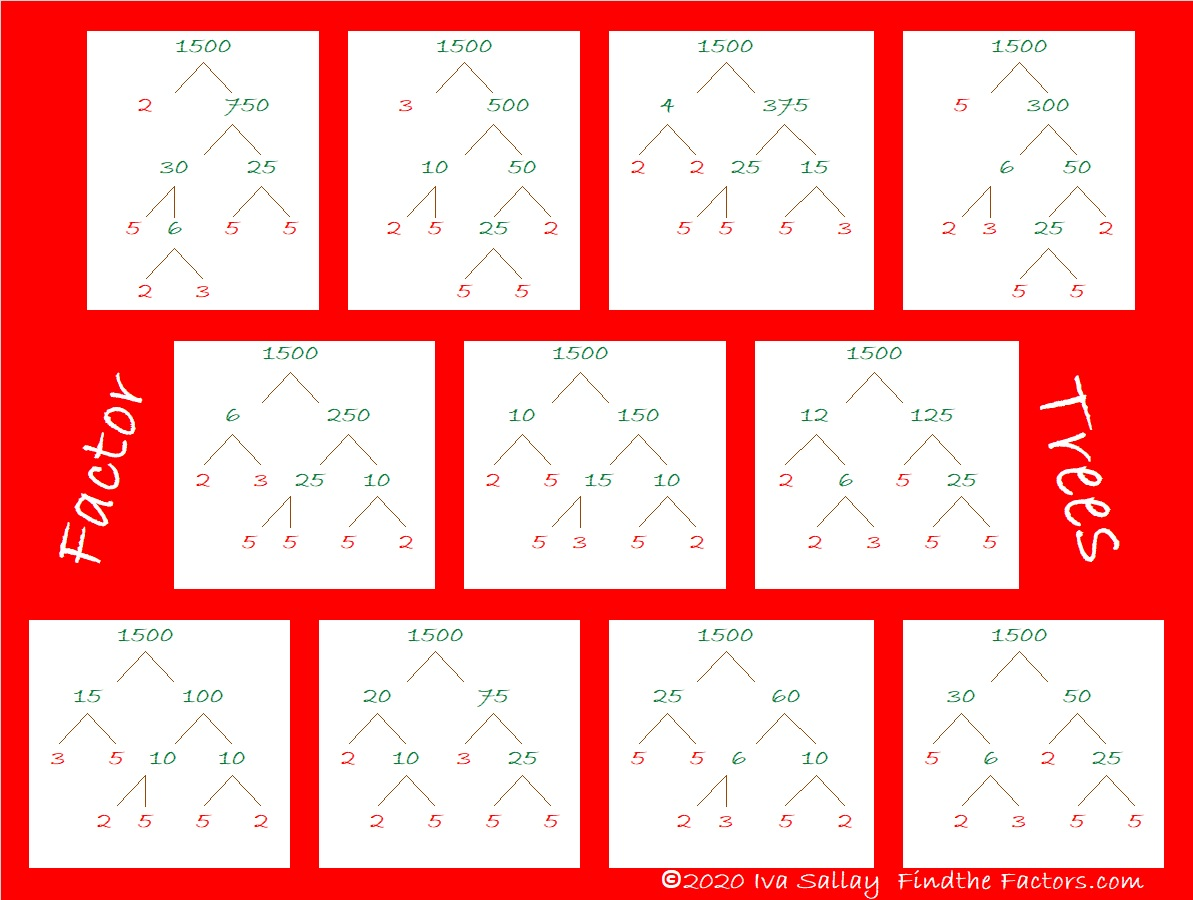### Other Facts to Celebrate about 1500:

Oeis.org tells us that  (5+1) × (5+5) × (5+0) × (5+0) = 1500.

1500 is the hypotenuse of THREE Pythagorean triples:
420-1440-1500, which is (7-24-25) times 60,
528-1404-1500, which is 12 times (44-117-225),
900-1200-1500, which is (3-4-5) times 300.

# 1470 Can You Find Factor Pairs That Make Sum-Difference?

### Today’s Puzzles:

I bet you can find a factor pair of 30 that adds up to 13 as well as another factor pair of 30 that subtracts to give you 13.

If you can solve that simple puzzle, then you will be able to solve the puzzle next to it. Even though 1470 has 12 different factor pairs, you don’t have to worry too much about them: All of the answers to the second puzzle are just _____ times the answers to the first puzzle! (And 1470 is _____² times 30.)Likewise, don’t get scared off with this next set of puzzles one of which wants you to find the factor pairs of 518616 that add up or subtract to 1470. Crazy, right? Again, if you can solve the first puzzle in the set, and if you can multiply a 3-digit number by a 1-digit number, you can easily solve the second puzzle because the answers are just _________ times the answers to the first puzzle in the set! (And 518616 is merely _________² times 6.)If you need more help than what I’ve already said, scroll down to the factor trees for 1470. I selected those particular trees for a reason!

### Factors of 1470:

• 1470 is a composite number.
• Prime factorization: 1470 = 2 × 3 × 5 × 7 × 7, which can be written 1470 = 2 × 3 × 5 × 7²
• 1470 has at least one exponent greater than 1 in its prime factorization so √1470 can be simplified. Taking the factor pair from the factor pair table below with the largest square number factor, we get √1470 = (√49)(√30) = 7√30
• The exponents in the prime factorization are 1, 1, 1, and 2. Adding one to each exponent and multiplying we get (1 + 1)(1 + 1)(1 + 1)(2 + 1) = 2 × 2 × 2 × 3 = 24. Therefore 1470 has exactly 24 factors.
• The factors of 1470 are outlined with their factor pair partners in the graphic below.### A Few Factor Trees for 1470:### More Facts about the Number 1470:

1470 is the average of 14² and 14³. That simple fact makes 1470 the 14th Pentagonal Pyramidal Number.

1470 is the hypotenuse of a Pythagorean triple:
882-1176-1470 which is (3-4-5) times 294

Hmm… that same factor pair showed up again!

# 210 and Level 4

### Today’s Puzzle:

Excel file of puzzles and previous week’s factor solutions: 10 Factors 2014-08-18

### Factors of 210:

• 210 is a composite number and a triangular number.
• Prime factorization: 210 = 2 x 3 x 5 x 7, so 210 is the product of the first four prime numbers!
• The exponents in the prime factorization are 1, 1, 1, and 1. Adding one to each and multiplying we get (1 + 1)(1 + 1)(1 + 1)(1 + 1) = 2 x 2 x 2 x 2 = 16. Therefore 210 has 16 factors.
• Factors of 210: 1, 2, 3, 5, 6, 7, 10, 14, 15, 21, 30, 35, 42, 70, 105, 210
• Factor pairs: 210 = 1 x 210, 2 x 105, 3 x 70, 5 x 42, 6 x 35, 7 x 30, 10 x 21, or 14 x 15
• Since 210 has no square factors, √210 cannot be simplified. √210 ≈ 14.491### Sum-Difference Puzzles:

210 has eight factor pairs. One of those factor pairs adds up to 29, and another one subtracts to 29. Another factor pair adds up to 37, and a different one subtracts to 37. Thus, 210 produces two different Sum-Difference Puzzles!210 is the smallest number that makes sum-difference two different ways!

### More about the Number 210:

210 is the hypotenuse of a Pythagorean triple:
126-168-210, which is (3-4-5) times 42.

Because 20 + 1 = 21 and (20/2) x 21 = 210, we know that 1 + 2 + 3 + 4 + 5 + 6 + 7 + 8 + 9 + 10 + 11 + 12 + 13 + 14 + 15 + 16 + 17 + 18 + 19 + 20 = 210, thus it is the 20th triangular number.

### A Logical Way to Solve Today’s Puzzle:# 180 and Level 1

### Today’s Puzzle: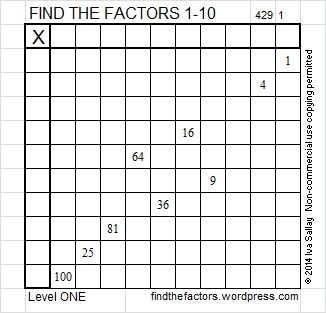Excel file of puzzles and previous week’s factor solutions: 10 Factors 2014-07-21

### Factors of 180:

 180 is a composite number. Factor pairs: 180 = 1 x 180, 2 x 90, 3 x 60, 4 x 45, 5 x 36, 6 x 30, 9 x 20, 10 x 18, or 12 x 15 Factors of 180: 1, 2, 3, 4, 5, 6, 9, 10, 12, 15, 18, 20, 30, 36, 45, 60, 90, 180 Prime factorization: 180 = 2 x 2 x 3 x 3 x 5 which can be written 180 = (2^2) x (3^2) x 5### Sum-Difference Puzzle:

180 has nine factor pairs. One of the factor pairs adds up to 41, and another one subtracts to 41. If you can identify those two factor pairs, then you can solve this puzzle!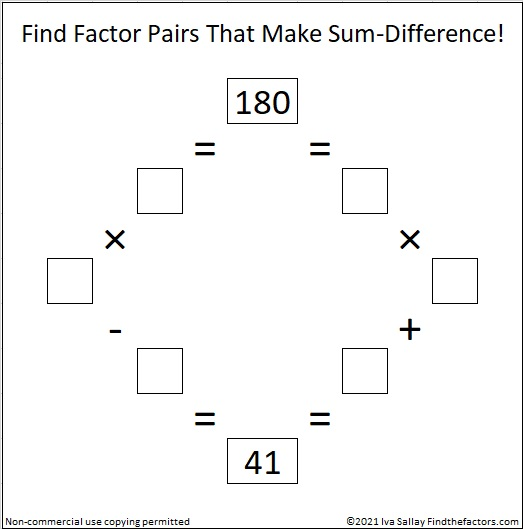### More about the Number 180:

There are 180 degrees in a triangle, and a straight angle is 180 degrees.

180 is the hypotenuse of a Pythagorean triple:
108-144-180, which is (3-4-5) times 36.

### Solution to This Week’s Puzzle:# 150 and Level 3

### Today’s Puzzle: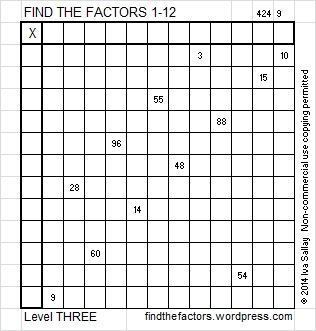### Factors of 150:

Excel file of puzzles and previous week’s factor solutions: 12 Factors 2014-06-16

 150 is a composite number. Factor pairs:150 = 1 x 150, 2 x 75, or 3 x 50, 5 x 30, 6 x 25, or 10 x 15 Factors of 150: 1, 2, 3, 5, 6, 10, 15, 25, 30, 50, 75, 150 Prime factorization: 150 = 2 x 3 x 5 x 5, which can also be written 150 = 2 x 3 x (5^2)### Sum-Difference Puzzle:

6 has two factor pairs. One of those factor pairs adds up to 5, and the other one subtracts to 5. Can you place those factors in the proper boxes to complete the first puzzle?

150 has six factor pairs. One of those factor pairs adds up to 25, and another one subtracts to 25. If you can identify those factor pairs, then you can complete the second puzzle!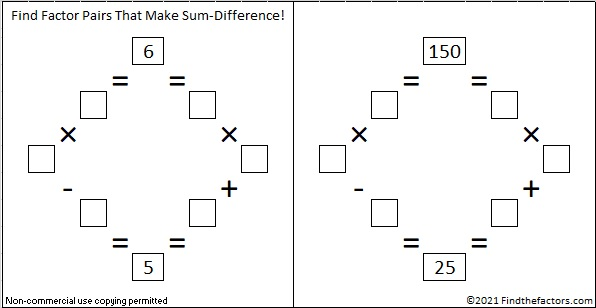The second puzzle is really just the first puzzle in disguise.  Why would I say that?

### A Logical Way to Solve Today’s Puzzle:

A Logical Approach to FIND THE FACTORS: Find the column or row with two clues and find their common factor. Write the corresponding factors in the factor column (1st column) and factor row (top row).  Because this is a level three puzzle, you have now written a factor at the top of the factor column. Continue to work from the top of the factor column to the bottom, finding factors and filling in the factor column and the factor row one cell at a time as you go.